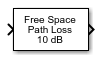# Free Space Path Loss

Apply free space path loss to complex signal

• Library:
• Communications Toolbox / RF Impairments

•## Description

The Free Space Path Loss block applies a free space path loss to a complex signal. The block simulates the loss of signal power due to the distance between the transmitter and receiver. The Mode parameter indicates whether you specify the loss in decibels or as a computation that is based on distance and the RF signal frequency.

## Ports

### Input

expand all

Complex signal, specified as a scalar or column vector.

Data Types: `double` | `single`
Complex Number Support: Yes

### Output

expand all

Output signal, returned as a scalar or column vector. This output is the same dimension and data type as the input signal.

## Parameters

expand all

Loss calculation mode, specified as one of these options.

• `Decibels` — The loss is specified using the Loss (dB) parameter.

• `Distance and Frequency` — The loss is computed using the Distance (km) and Carrier frequency (MHz) parameters.

Power loss in decibels, specified as a scalar. The decibel amount shown on the block icon is rounded for display purposes only.

#### Dependencies

To enable this parameter, set the Mode parameter to `Decibels`.

Distance between the transmitter and receiver in kilometers, specified as a scalar.

#### Dependencies

To enable this parameter, set the Mode parameter to `Distance and Frequency`.

Carrier frequency in megahertz, specified as a scalar.

#### Dependencies

To enable this parameter, set the Mode parameter to `Distance and Frequency`.

## Block Characteristics

 Data Types `double` | `single` Multidimensional Signals `no` Variable-Size Signals `no`

## Algorithms

The free-space path loss, L, in decibels is:

L = 20log10(4πR/λ).

This formula assumes that the target is in the far-field of the transmitting element or array. In the near-field, the free-space path loss formula is not valid and can result in a loss smaller than 0 dB, equivalent to a signal gain. For this reason, the loss is set to 0 dB for range values R ≤ λ/4π.

## Version History

Introduced before R2006a